Examples from Takashi Kojima's book "Advances Abacus, Theory and Practice"
(All examples are taken from the chapter "Other Methods of Multiplication.")

Multiplication by Complementary Numbers

Multiplication can often be simplified by using the complement of a multiplier. For instance, take the problem 26 x 98. The 26 is multiplied by 100 becoming 2,600. From this 26 x 2 is subtracted. In this way the answer 2,548 is obtained. The 2 is, of course, the complement of 98 with respect to 100. This method of computation is better than the ordinary method of multiplication when the the multiplier is  a number a little smaller than 100 or 1,000 etc., such as 97 or 996 etc.

Example 1:  26 x 98 = 2,548

Step 1: Set the multiplicand 26 on DE and on A, set 2, the complement of 98 with respect to 100 (Fig 1). When the multiplier is a two-figure number, the multiplicand is regarded as having been multiplied by 100. So in this problem the unit rod of the product shifts to G.

Fig. 1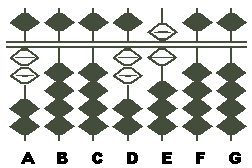Step 2: Multiplying the 2 on A by the 6 on E, subtract the product 12, which is to be set on FG, from the 6 on E. This leaves 2,588 on DEFG (Fig. 2)

Fig. 2Step 3: Multiplying the 2 on A by the 2 on D, subtract the product 4 from the 8 on F. This leaves 2,548 on DEFG (Fig.3), the answer.

Fig. 3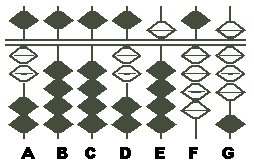Example 2:  A manufacturer wishes to purchase a piece of machinery priced at \$7,250 on which there is a discount of 4% for cash. Find the price he pays in cash. The answer can be found by the following simplified multiplication.

7,250 x 0.96
=7,250 x (1 - 0.04)
=7,250 - (7,250 x 0.04)
=6,960

Step 1: Set the multiplicand 7,250 on DEFG and on A, set the 4 of 0.04, which is the complement of 0.96 with respect to 1. (Fig.1)

Fig. 1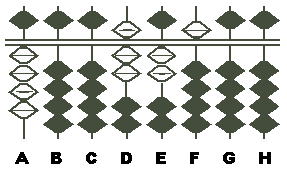NOTE:  On the board you have this problem: 7,250 - (7,250 x 0.04). In this problem, the actual multiplier is 0.96, i.e., a decimal with it's first significant digit in the tenths place. So you may consider that the answer is obtained by keeping the multiplicand as it is and subtracting from it 7,250 x 0.04. You should note that when the multiplier is a decimal with its first significant digit in the tenths place, the unit rod of the product remains that of the multiplicand.

Step 2: Multiplying the 4 on A by the 5 on F, subtract the product 20, which is to be set on GH, from the 5 on F. This leaves 7,248 on DEFG. (Fig.2)

Fig. 2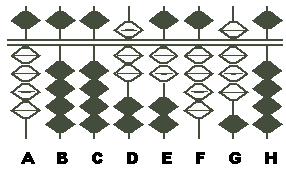NOTE: As the 5 on F is in the tens place of the multiplicand, the product 20 must be set on GH.

Step 3: Multiplying the 4 on A by the 2 on E, subtract the product 8 from the 8 on G. This leaves 724 on DEF. (Fig.3)

Fig.3Step 4: Multiplying the 4 on A by the 7 on D, subtract the product 28 from 724 on DEF. This leaves 6,960 on DEFG (Fig.4).  The answer is \$6,960

Fig.4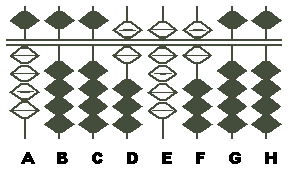- As written by Takashi Kojima
from his book, "Advances Abacus, Theory and Practice"
Publisher: Charles E. Tuttle, 1954

P.S. One more example can be found by following the link below;

Comp2.htm